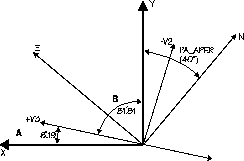[Top] [Prev] [Next] [Bottom]

33.5 Converting FOS X,Y to Spacecraft V2,V3

Although the conversion of telescope offsets from the FOS (x,y) reference frame to the telescope (V2,V3) reference frame is provided in the FOS paper products for all acquisition slews, you may occasionally need to calculate this rotation for other purposes. As noted in Chapter 29, the FOS (x.y) coordinate system is defined so that the x-axis is parallel to the diode array and the y-axis is perpendicular to the diode array. Further, the (x.y) coordinate frame is rotated with respect to the telescope (V2,V3) coordinate frame. Figures 33.2 and 33.3 show the coordinate axes for both detectors before COSTAR was installed. The (V2,V3) axes were rotated 180° by the introduction of COSTAR.

Figure 33.3: Pre-COSTAR Orientation of FOS/RD (PA_APER=40°)From the figures above we see that the coordinate transformation for FOS/BL is given by:

33.5.1 Converting X,Y to RA, Dec

The conversion of an (x,y) offset to an (RA,Dec) offset is, of course, an additional simple rotation added to the expressions in section "Converting FOS X,Y to Spacecraft V2,V3" on page 33-8. The position angle of the aperture (PA_APER) is the angular orientation of the +y-axis of the aperture measured in degrees east of North. PA_APER is given in the FOS paper products and in science data headers.

[Top] [Prev] [Next] [Bottom]

stevens@stsci.edu